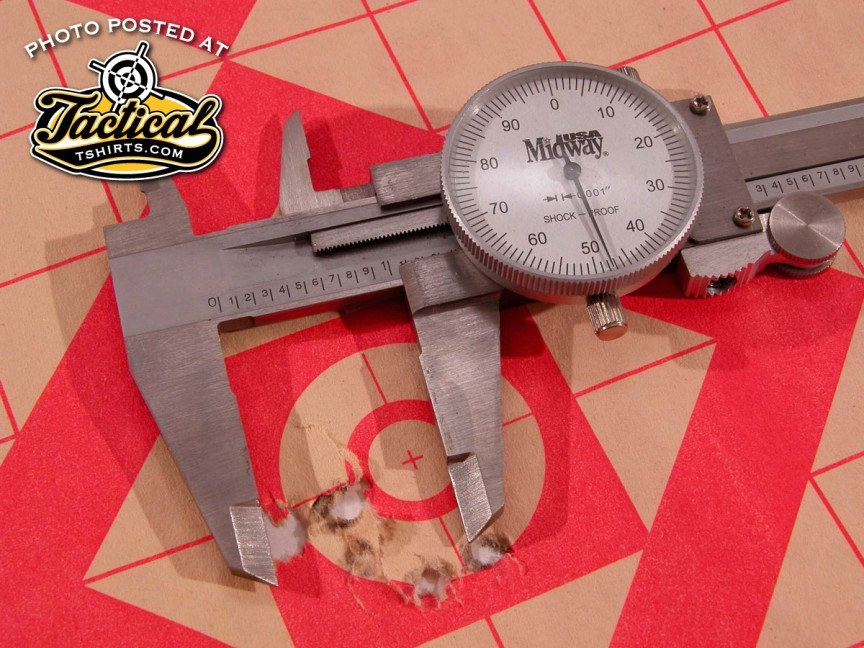# Meaning of MOA — by Scott Mayer

Meaning of MOA?

You’ll often hear gun accuracy expressed in terms of “MOA.” For example, Weatherby Range Certified rifles are guaranteed to shoot “sub-MOA,” but do you know what MOA means?

MOA stands for “Minute Of Angle,” which is a unit of angular measurement. When the accuracy of a gun is given in minutes of angle, it is possible for a person to know what size groups the gun shoots within its effective range.

There are 360 degrees in a circle, and 60 minutes of arc in each degree, totaling 21,600 minutes of angle in a complete circle. Imagine the shooter is at the center of a circle, and that the target is on the edge of that circle. The distance, or range, from the shooter to the target is also the radius [R] of the circle. For any given range or radius [R], one minute is equal to 2πR÷21,600.

If the range is 100 yards, that distance is equal to 3,600 inches. When we plug 3,600 in for R in our formula, the result is (2)(3.1416)(3,600)÷21,600 = 1.0472 inches. Therefore, at 100 yards, one MOA is equal to 1.0472 inches. The formula works for any range. If the range is 500 yards, one MOA equals 5.2360 inches.MOA is a unit of angular measurement equal to 1.0472 inches at 100 yards. For all intents and purposes, you can call that one inch. The difference between a true MOA and the inch per 100 yard measure only becomes obvious at extreme range.

Many shooters equate one MOA to one inch at 100 yards, 2 inches at 200 yards, and so on. Often, they will call a gun that shoots one-inch groups at 100 yards a “one-MOA gun.” Since targets are usually on an inch grid, and sight adjustments in fractions of an inch, the difference between “true” minutes of angle and “shooters'” minutes of angle is academic.

Scott Mayer

www.tacticaltshirts.com

“Shooting Guns & Having Fun”

Latest posts by Scott Mayer (see all)